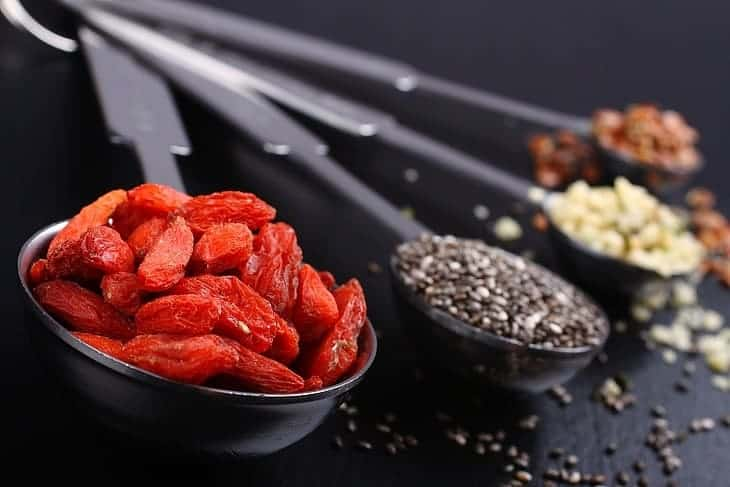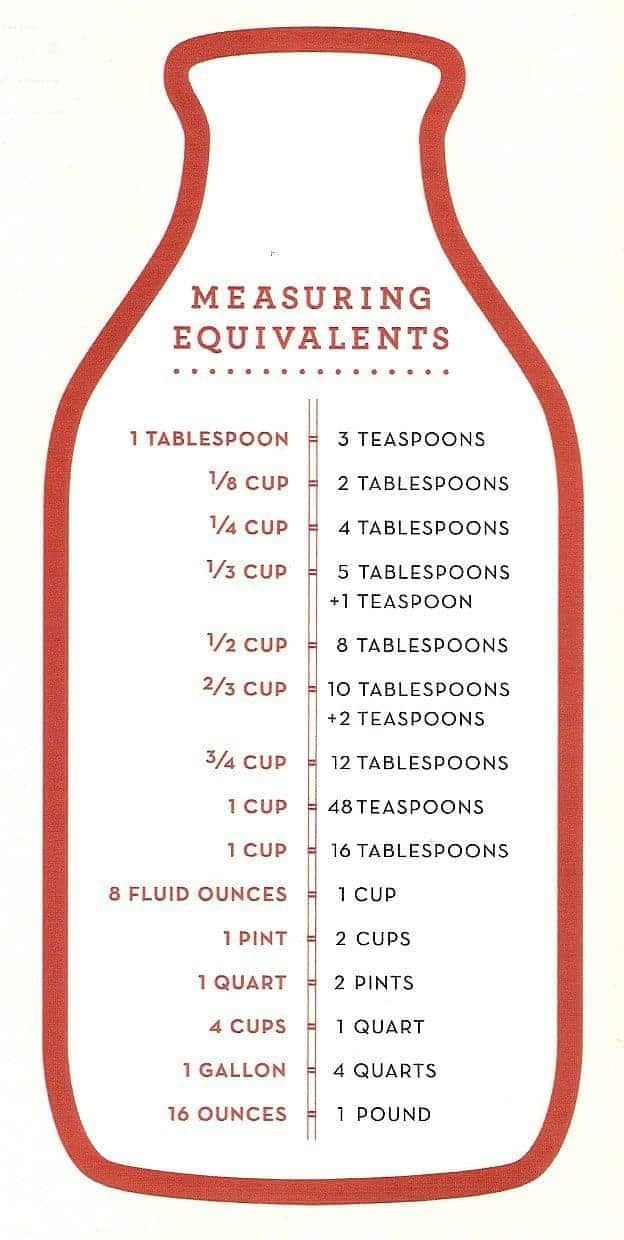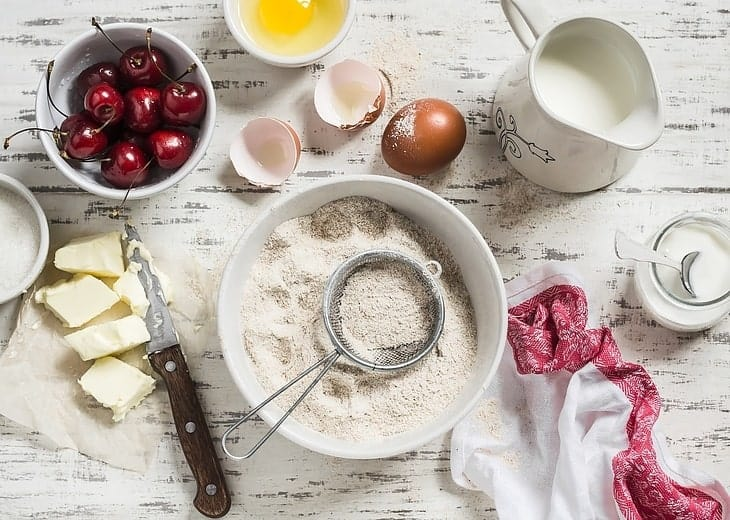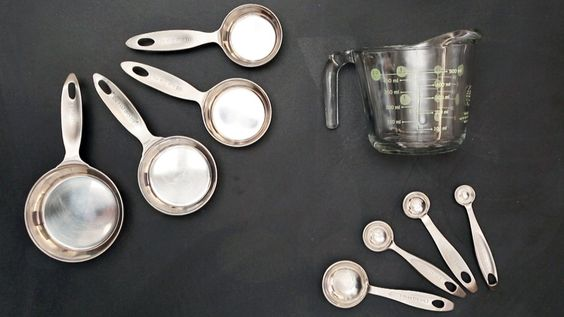# The Truth About How Many Tablespoons In 2/3 Cup Might Surprise You

It happens all the time: you're in the kitchen and you have to figure out how many tablespoons there are in 2/3 cup of an ingredient.

In America, most servings are calculated by volume rather than weight. This means you don't have to worry about how much something weighs to get your answer, but converting the measurement to grams can allow you to be more precise.

This can be a frustrating problem because you probably don't want to solve complex math problems while cooking. Lucky for you, we've created this guide to help you easily get the information you need.

The Truth About How Many Tablespoons In 2/3 Cup Might Surprise You

## Solving Tablespoon WoesFlour or water? Peanut butter or jam? The answer for how many tablespoons there are in 2/3 of a cup is easier to figure out than you may think.

Since a tablespoon and a cup are both measures of volume and not weight, the answer is going to be the same no matter what substance you're using. Check out this helpful video below to learn the basics about measuring dry or liquid ingredients.

## What's In A Cup?AllRecipes.com has a simple chart for determining the appropriate number of tablespoons for any conversion.

By using their chart, you can easily see that there are 16 TBSP (tablespoons) in 1 cup. This means there are 10 2/3 TBSP in 2/3 of a cup.

​To measure this out, you can either eyeball it, or you can use 10 TBSP + 2 teaspoons (TSP) to get 2/3 cups since there are 3 TSP in a TBSP. In decimal form, this works out to 10.67 TBSP for every 2/3 cup.

When measuring with tablespoon, use a set of tablespoons that is suitable for both dry and liquid ﻿﻿﻿﻿ingredients. The 1EasyLife H742 Stainless Steel set of measuring spoons provides a product that will give you consistent results every time.

## Using A Scale To Measure Tablespoons

Since measuring out 10 2/3 tablespoon could pose some difficulty, you can convert your answer to grams. To do this, you'll need a scale. Use a scale like the Etekcity Digital Kitchen Food Scale for the most accurate results.

• Turn the scale on and tare to zero.
• Choose the gram function.
• Add 1 TBSP of your desired substance to the scale's cup.
• Multiply that number by 10.67.

This answer provides an exact measurement in grams for the equivalent number of tablespoons in 2/3 of a cup. Fill the scale until you get the precise amount of the ingredient you need.

​For example, 1 TBSP of water = 14.79 grams. Multiply that number by 10.67, and you get a total 158 grams after rounding up to a whole number.

Measuring out 158 grams of water with a scale can be easier than measuring 10 tablespoons and 2 teaspoons of water. If you like, use an online calculator to figure out the exact amount of grams in each tablespoon of a liquid.

## Scaling A RecipeIf you need to scale a ﻿recipe﻿, you can quickly perform calculations by using the conversion you figured out for tablespoons to grams. Remember that every ingredient that you convert from tablespoons to grams must be converted by itself because some substances will weigh more than others.

For example, a TBSP of butter is going to have a different weight than a TBSP of milk. You can use online calculators to figure out the conversion of most products, but it's best to figure out the conversion on your own.

If you want a little help scaling your recipes to avoid doing all of the math yourself, consider using a tool like the Kitchen Calc Pro to figure out your recipes.

This little calculator performs all manner of calculations for you. You'll be able to quickly adjust a recipe, perform conversions between U.S. and foreign weights and measures, and determine proportions to adjust the servings.

The company even has a video to help you learn how to use the calculator.

## Calculating Fractions

Once you know how many tablespoons are in a cup, you can make the conversion to fractions.

If you want to know how many tablespoons are in 2/3 of a cup, you can simply:

• Divide the total number of tablespoons in a cup by 3.
• Multiply that value by 2 to figure out how many tablespoons are in 2/3 cup.

### Using the gram conversion.

An easier way to do this is to use the gram conversion. We've established that 1 TBSP of water = 14.79 grams. When you multiply that number by 16 (amount of TBSP in a cup), you'll arrive at 236.64 grams of water in a single cup.

Now, all you have to do is multiply the number by 0.67 (rounded decimal of 2/3). You'll find that there are about 158 grams in 2/3 of a cup.

If you compare the earlier math we did, you'll find that the two methods of calculation don't provide the exact same numbers, but this is because we're rounding up the decimals so we don't have to deal with complex numbers.

In the first method, 14.79 x 10.67 = 157.8093. In the second example, where you multiply 14.79 by 16 and then multiply that by 0.67, you'll get an answer of 158.5488.marthastewart.com

If this is too much math, simply use a good calculator that figures out the conversions for you, and you'll be ready to make your delicious recipes.

While you can also download an app for your smartphone to perform the calculations, you probably don't want to worry about getting your smartphone in the thick of things when the ingredients start flying. Kitchen calculators provide protection against water and almost 2,000 hours of use on a single battery.

## Conclusion

Overcome any conversion with the right calculator by your side. If you only need the most basic functions, choose the Kitchen Calc Pro calculator.

For more intense recipes, upgrade to the KitchenCalc Pro Master Chef Edition.